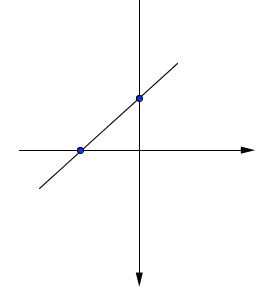### Home > GC > Chapter Ch1 > Lesson 1.1.5 > Problem1-43

1-43.

Using graph paper, draw a pair of $xy$-axes and scale them for $-6 \leq x \leq 6$ ($x$-values between and including $−6$ and $6$) and $−10 \leq y \leq 10$ ($y$-values between and including $−10$ and $10$). Complete the table below, substituting the $x$-values (inputs) to find the corresponding $y$-values (outputs) for the rule $y = x + 2$. Plot and connect the resulting points...Homework Help ✎

 $x$ (input) $−4$ $−3$ $−2$ $−1$ $0$ $1$ $2$ 3 4 $y$ (output)

Line has a $y$-intercept of $(0, 2)$ and an $x$-intercept of $(− 2,0)$.

$\left. \begin{array} { | c | c | c | c | c | c | c | c | } \hline x ( \text { input } ) & { - 4 } & { - 3 } & { - 2 } & { - 1 } & { 0 } & { 1 } & { 2 } & { 3 } & { 4 } \\ \hline y ( \text { output } ) & { - 2 } & { - 1 } & { 0 } & { 1 } & { 2 } & { 3 } & { 4 } & { 5 } & { 6 } \\ \hline \end{array} \right.$